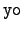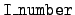Next: The kinematics directories Up: The format for mcf.bin' Previous: The format for mcf.bin'   Contents

## The segments of a kinematics directory

The kinematics directories differ considerably in form. This is because different kinds of data may be given, depending on the kinematics type of the reaction. Any kinematics directory is, however, built up of some number of the following segments. Note that all data-location entries are relative to the start of the data block for the target. This reference location is given in the target directory.

Identification of secondary particle:
The following four numbers.

yo:
The particle-number identifier.
ZA:
The secondary particle identified as 1000*Z + A.
Excitation:
The excitation level of the secondary particle.
Mass:
The atomic mass of the particle.

Scatterer identification:
This block of three numbers is given for reactions involving the scattering of photons.

ZA:
The convention is that an electron has ZA = - 9.
yo:
For an electron we have= 9.
Mass:
The atomic mass of the scatterer.

Angular bins:
For= 1 data we have four numbers.

Number of bin edges:
The number of the equiprobable angular bin boundaries.

Number of energies:
The number of incident energies for which data is given.

Bin location:
The location of the first angular bin for the first (smallest) incident energy. For each incident energy the bin edges are ordered by increasing cosine.

Energy location:
The location of the list of incident energies. The energies are in increasing order.

Energy-angle bins:
For= 3 data we have the following set of numbers.

Number of energies:
The number of incident energies for which data is given.

Energy location:
The location of the list of incident energies.

Bin locations:
We have a block of numbers, one for each incident energy. The data is organized as: the edge of an equiprobable cosine bin followed by the corresponding equiprobable energy bins for the secondary particle. For each incident energy the number given in this segment of the kinematics directory is the location of the first equiprobable cosine bin edge. The cosines are in increasing order, as are the energy bin edges.

Number of cosines:
The number boundary points for the equiprobable angular bins.

Number of energies for secondary:
The number of equiprobable secondary energy bin boundaries.

Block length:
The length of a data block corresponding to the bin locations above. This number is equal to the product,

(number of cosines)*(1 + number of secondaryI>energies).

Energy bins:
For= 4 data we have four numbers.

Number of bin edges:
The number of equiprobable secondary energy bin boundaries.

Number of energies:
The number of incident energies for which data is given.

Bin location:
The location of the first secondary energy bin for the first (smallest) incident energy. For each incident energy the secondary energy bins are in increasing order.

Energy location:
The location of the list of incident energies. The incident energies are in increasing order.

Multiplicity:
For= 7 and= 9 data we have either one or five numbers.

Multiplicity:
If the multiplicity of the secondary particle is independent of the energy of the incident particle, it is given here as a real number, and this segment contains no other numbers. A zero here indicates that the multiplicity is energy dependent, and the following four numbers are also present.

Data length:
The number of multiplicity-data entries. There is one entry for each energy group.

Data location:
The location of the multiplicity data.

gp_start:
The index of the first energy group for which data is given. This corresponds to the energy threshold.

gp_end:
The index of the last energy group for which data is given.

Energy deposition:
For= 10 data we have the following four numbers.

Data length:
The number of energy-deposition entries. There is one entry for each energy group.

Data location:
The location of the energy-deposition data.

gp_start:
The index of the first energy group for which data is given. This corresponds to the energy threshold.

gp_end:
The index of the last energy group for which data is given.

Photon scattering:
For= 941 or= 942 data we the following three numbers.

Interpolation type:Next: The kinematics directories Up: The format for mcf.bin' Previous: The format for mcf.bin'   Contents